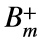# Problem 45831. Compute the Bernoulli numbers

The Bernoulli numbers appear in several applications in number theory, including expressions for sums of powers and certain values of the Riemann zeta function. For each index m, the Bernoulli numbers have two values, one denoted with a plus superscript and one denoted with a minus superscript.
For m = 0, 1, 2, 3, 4, the "plus" values are= 1, 1/2, 1/6, 0, and -1/30, respectively. The "minus" values are the same, except for m = 1, for which the value is -1/2.
Compute the two values of the Bernoulli numbers for the given index. Round to seven places after the decimal.

### Solution Stats

34.29% Correct | 65.71% Incorrect
Last Solution submitted on Apr 21, 2023

### Community Treasure Hunt

Find the treasures in MATLAB Central and discover how the community can help you!

Start Hunting!